Foxtable(狐表)用户栏目专家坐堂 → 甘特图实际开始日期和结束日期

共有1713人关注过本帖树形打印复制链接

# 主题：甘特图实际开始日期和结束日期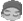nuoyan88
1楼 | 信息 | 搜索 | 邮箱 | 主页 | UC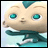加好友发短信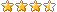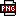此主题相关图片如下：1.png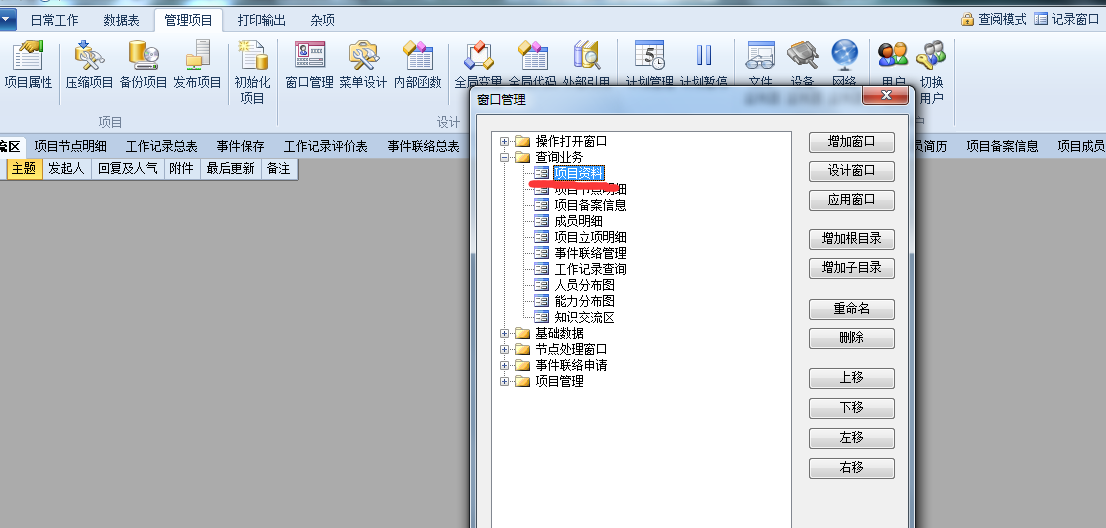此主题相关图片如下：2.png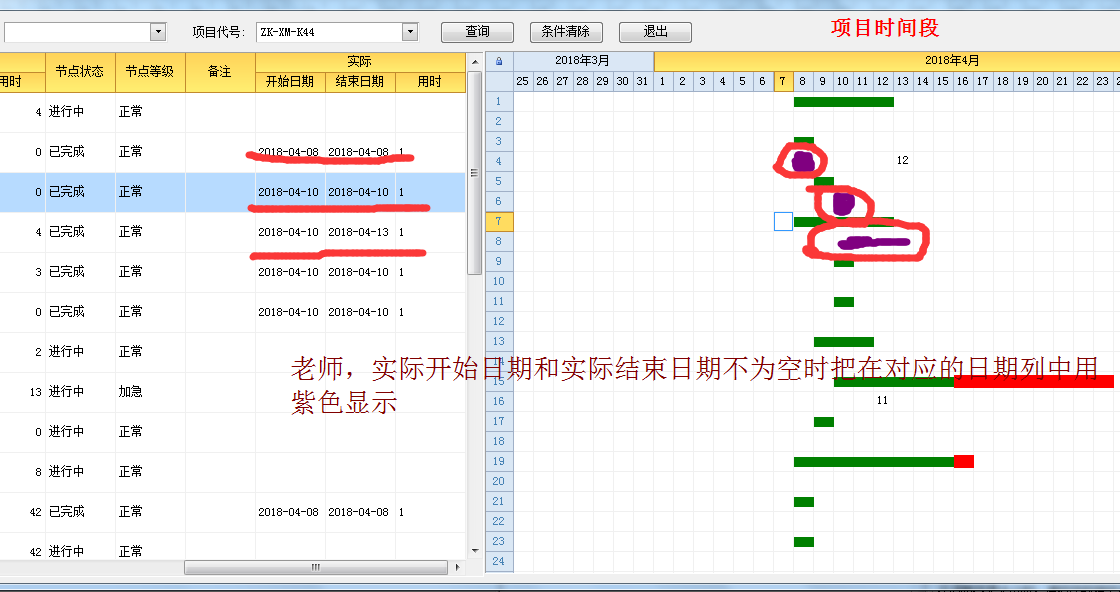下载信息  [文件大小：   下载次数： ]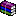点击浏览该文件:规划管理系统0417.rar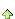2楼 | 信息 | 搜索 | 邮箱 | 主页 | UC加好友发短信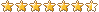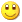Post By：2018/4/16 21:07:00 [只看该作者]

 drawcell事件If DrawGannt    If e.Row.Index Mod 2 = 0 Then        Dim r As Row  = Tables("项目资料_Table1").Rows(e.Row.Index/2)        Dim dt1 As Date = r("计划_开始日期")        Dim dt2 As Date = r("计划_结束日期")        Dim sdt1 As Date = r("实际_开始日期")        Dim sdt2 As Date = r("实际_结束日期")        Dim dt As Date = e.Col.Name.Replace("年","-").Replace("月_","-")        If dt>=dt1 AndAlso dt<=dt2 OrElse dt>=sdt1 AndAlso dt<=sdt2 Then            e.StartDraw()            If dt>=sdt1 AndAlso dt<=sdt2                e.Graphics.FillRectangle(Brushes.Purple,e.x ,e.y + 5, e.Width, e.Height - 10)            Else                If dt < Date.Today Then                    e.Graphics.FillRectangle(Brushes.Green,e.x ,e.y + 5, e.Width, e.Height - 10)                Else                    e.Graphics.FillRectangle(Brushes.Red,e.x ,e.y + 3, e.Width, e.Height - 7)                End If            End If            e.EndDraw()        End If    Else        Dim dt As Date = e.Col.Name.Replace("年","-").Replace("月_","-")        Dim r As Row  = Tables("项目资料_Table1").Rows(e.Row.Index\2)        Dim fdr As DataRow = DataTables("工作记录总表").find("计划日期=#" & dt & "# and 节点代号='" & r("节点代号") & "' and 项目代号='" & r("项目代号") & "'")        If fdr IsNot Nothing Then            e.text = fdr("总用时")        End If    End IfEnd Ifnuoyan88
3楼 | 信息 | 搜索 | 邮箱 | 主页 | UC加好友发短信Post By：2018/4/16 21:54:00 [只看该作者]

 老师，计划_开始日期和计划_结束日期能不能不变动，还是按照之前的显示，实际_开始日期和实际_结束日期显示在对应的第二行可以吗？如图，谢谢此主题相关图片如下：1.png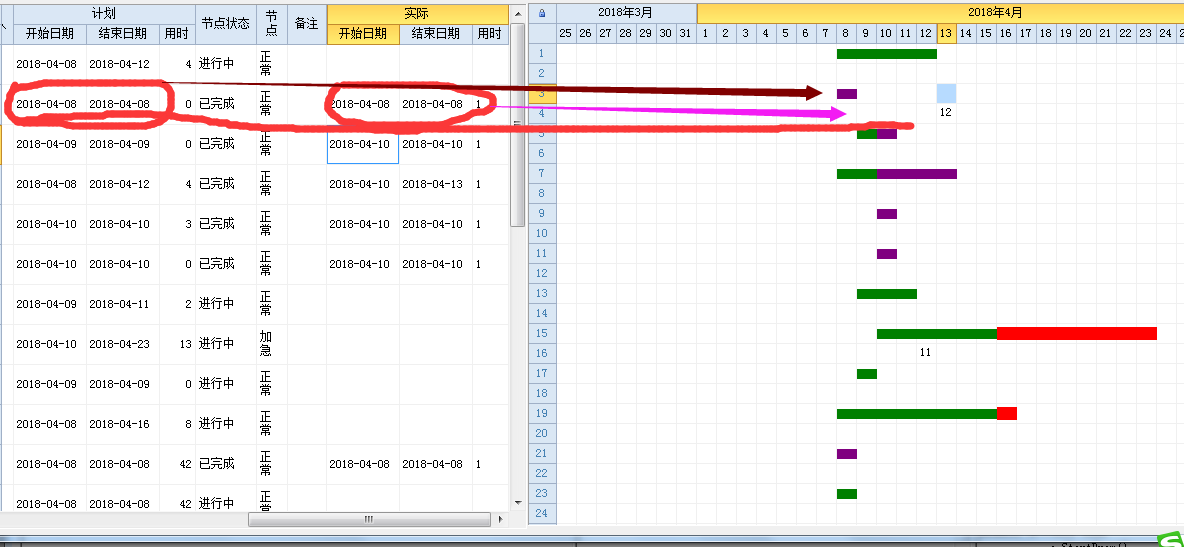4楼 | 信息 | 搜索 | 邮箱 | 主页 | UC加好友发短信Post By：2018/4/16 22:01:00 [只看该作者]

 增加一个判断，写到If e.Row.Index Mod 2 = 1 的条件中去nuoyan88
5楼 | 信息 | 搜索 | 邮箱 | 主页 | UC加好友发短信Post By：2018/4/16 22:09:00 [只看该作者]

 老师，实在不好意思，不知道怎么写？以下红色没有改对。 If DrawGannt    If e.Row.Index Mod 2 = 1 Then        Dim r As Row  = Tables("项目资料_Table1").Rows(e.Row.Index/2)        Dim dt1 As Date = r("计划_开始日期")        Dim dt2 As Date = r("计划_结束日期")        Dim sdt1 As Date = r("实际_开始日期")        Dim sdt2 As Date = r("实际_结束日期")6楼 | 信息 | 搜索 | 邮箱 | 主页 | UC加好友发短信Post By：2018/4/16 22:34:00 [只看该作者]

 If DrawGannt    Dim dt As Date = e.Col.Name.Replace("年","-").Replace("月_","-")    If e.Row.Index Mod 2 = 0 Then        Dim r As Row  = Tables("项目资料_Table1").Rows(e.Row.Index/2)        Dim dt1 As Date = r("计划_开始日期")        Dim dt2 As Date = r("计划_结束日期")                If dt>=dt1 AndAlso dt<=dt2 Then            e.StartDraw()            If dt < Date.Today Then                e.Graphics.FillRectangle(Brushes.Green,e.x ,e.y + 5, e.Width, e.Height - 10)            Else                e.Graphics.FillRectangle(Brushes.Red,e.x ,e.y + 3, e.Width, e.Height - 7)            End If            e.EndDraw()        End If    Else        Dim sdt1 As Date = r("实际_开始日期")        Dim sdt2 As Date = r("实际_结束日期")        If  dt>=sdt1 AndAlso dt<=sdt2 Then            e.StartDraw()            e.Graphics.FillRectangle(Brushes.Purple,e.x ,e.y + 5, e.Width, e.Height - 10)            e.EndDraw()        End If                Dim r As Row  = Tables("项目资料_Table1").Rows(e.Row.Index\2)        Dim fdr As DataRow = DataTables("工作记录总表").find("计划日期=#" & dt & "# and 节点代号='" & r("节点代号") & "' and 项目代号='" & r("项目代号") & "'")        If fdr IsNot Nothing Then            e.text = fdr("总用时")        End If    End IfEnd Ifnuoyan88
7楼 | 信息 | 搜索 | 邮箱 | 主页 | UC加好友发短信Post By：2018/4/16 22:43:00 [只看该作者]

 老师，请帮忙看看，谢谢此主题相关图片如下：1.png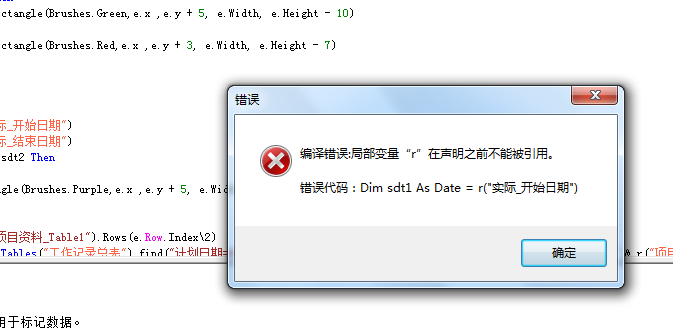8楼 | 信息 | 搜索 | 邮箱 | 主页 | UC加好友发短信Post By：2018/4/16 23:02:00 [只看该作者]

 这么明显的提示和简单的错误，自己学会分析一下原因和排错nuoyan88
9楼 | 信息 | 搜索 | 邮箱 | 主页 | UC加好友发短信Post By：2018/4/17 8:26:00 [只看该作者]

 老师，错误改好了，但是结果不对，是不是我哪里没有改对呢？谢谢 If DrawGannt    Dim dt As Date = e.Col.Name.Replace("年","-").Replace("月_","-")    If e.Row.Index Mod 2 = 0 Then        Dim r As Row  = Tables("项目资料_Table1").Rows(e.Row.Index/2)        Dim dt1 As Date = r("计划_开始日期")        Dim dt2 As Date = r("计划_结束日期")        If dt>=dt1 AndAlso dt<=dt2 Then            e.StartDraw()            If dt < Date.Today Then                e.Graphics.FillRectangle(Brushes.Green,e.x ,e.y + 5, e.Width, e.Height - 10)            Else                e.Graphics.FillRectangle(Brushes.Red,e.x ,e.y + 3, e.Width, e.Height - 7)            End If            e.EndDraw()        End If    Else        Dim s As Row  = Tables("项目资料_Table1").Rows(e.Row.Index/2)        Dim sdt1 As Date = s("实际_开始日期")        Dim sdt2 As Date = s("实际_结束日期")        If  dt>=sdt1 AndAlso dt<=sdt2 Then            e.StartDraw()            e.Graphics.FillRectangle(Brushes.Purple,e.x ,e.y + 5, e.Width, e.Height - 10)            e.EndDraw()        End If        Dim r As Row  = Tables("项目资料_Table1").Rows(e.Row.Index\2)        Dim fdr As DataRow = DataTables("工作记录总表").find("计划日期=#" & dt & "# and 节点代号='" & r("节点代号") & "' and 项目代号='" & r("项目代号") & "'")        If fdr IsNot Nothing Then            e.text = fdr("总用时")        End If    End IfEnd If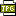此主题相关图片如下：3.jpg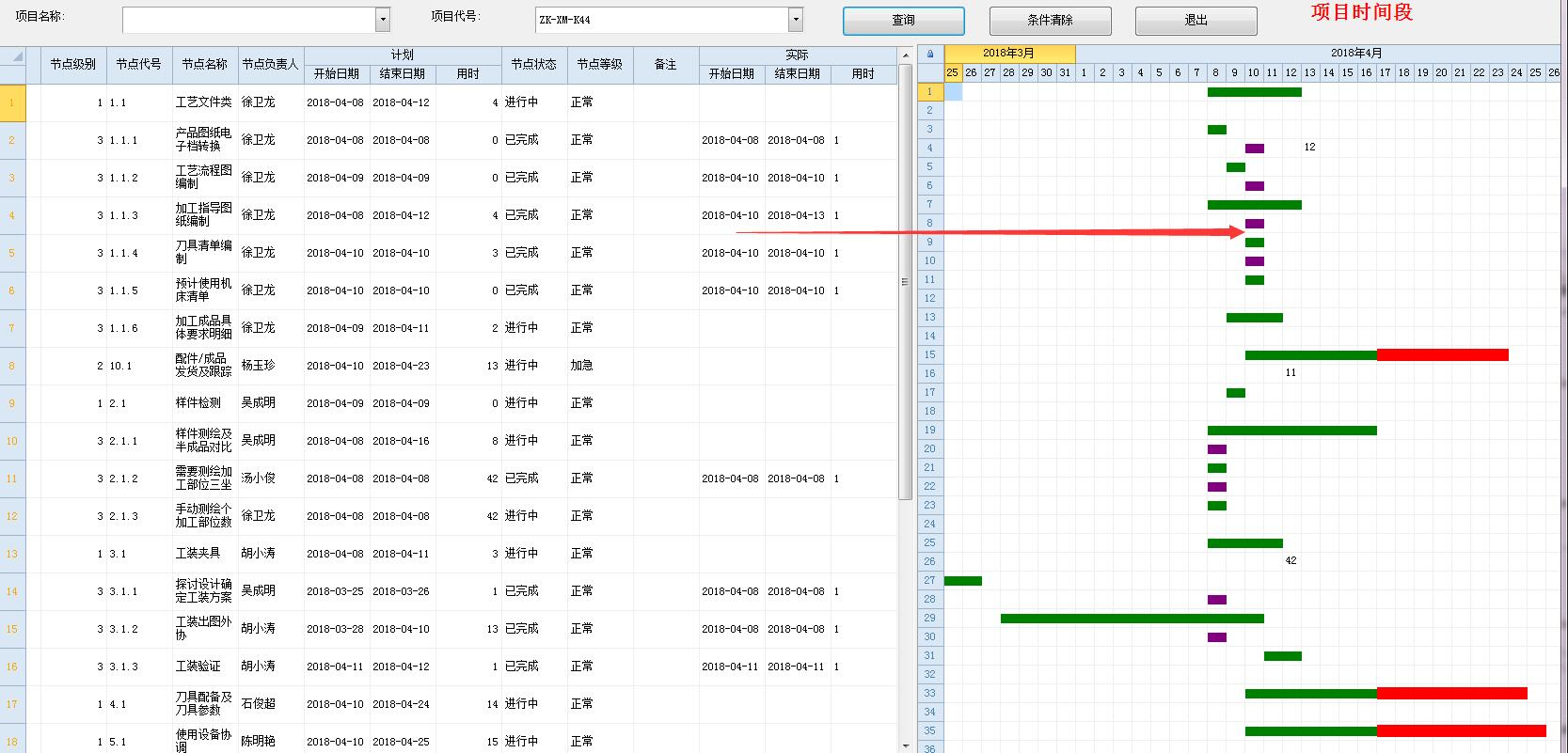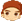10楼 | 信息 | 搜索 | 邮箱 | 主页 | UC加好友发短信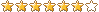Post By：2018/4/17 8:45:00 [只看该作者]

 改成这样测试           Dim s As Row  = Tables("项目资料_Table1").Rows(e.Row.Index/2)        Dim sdt1 As Date = s("实际_开始日期")        Dim sdt2 As Date = s("实际_结束日期")        If  dt>=sdt1 AndAlso dt<=sdt2 Then            e.StartDraw()            e.Graphics.FillRectangle(Brushes.Purple,e.x ,e.y + 5, e.Width, e.Height - 10)            e.EndDraw()        End If        'Dim r As Row  = Tables("项目资料_Table1").Rows(e.Row.Index\2)        'Dim fdr As DataRow = DataTables("工作记录总表").find("计划日期=#" & dt & "# and 节点代号='" & r("节点代号") & "' and 项目代号='" & r("项目代号") & "'")        'If fdr IsNot Nothing Then            'e.text = fdr("总用时")        'End If   如果可以，就用gdi把总用时也画上去 http://www.foxtable.com/webhelp/scr/1482.htm总数 12 1 2 下一页